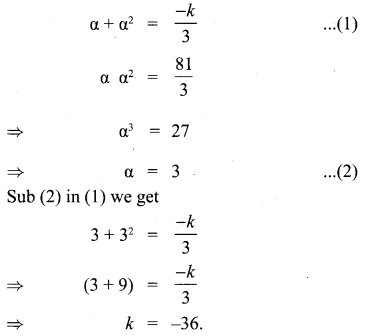# Samacheer Kalvi 10th Maths Solutions Chapter 3 Algebra Ex 3.14

## Tamilnadu Samacheer Kalvi 10th Maths Solutions Chapter 3 Algebra Ex 3.14

10th Maths Exercise 3.14 Solutions Question 1.
Write each of the following expression in terms of α + β and αβ.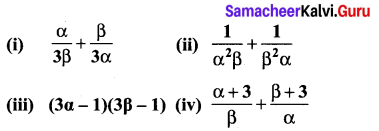Solution: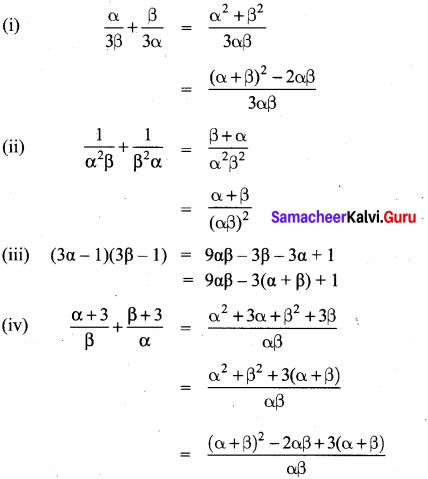Ex 3.14 Class 10 Samacheer Question 2.
The roots of the equation 2x2 – 7x + 5 = 0 are α and β. Without solving the root find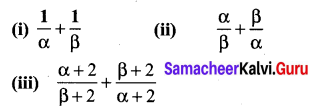Solution:
2x2 – 7x + 5 = x2 – $$\frac{7}{2} x+\frac{5}{2}$$ = 0
α + β = $$\frac{7}{2}$$
αβ = $$\frac{5}{2}$$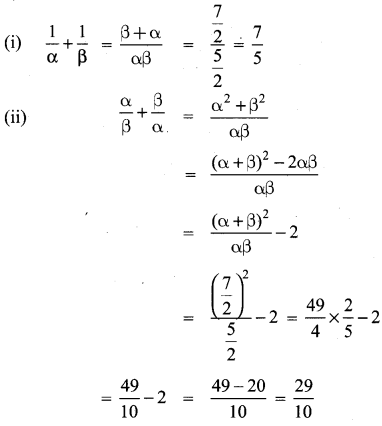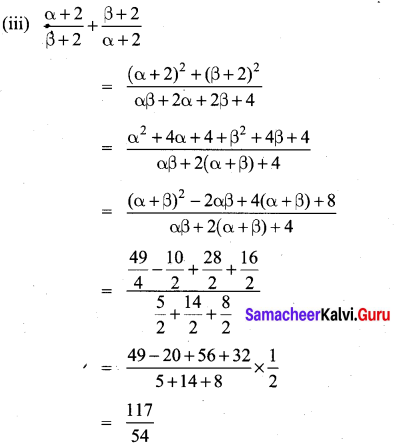10th Maths Exercise 3.14 Question 3.
The roots of the equation x2 + 6x – 4 = 0 are α, β. Find the quadratic equation whose roots are
(i) α2 and β2
(ii) $$\frac{2}{\alpha} \text { and } \frac{2}{\beta}$$
(iii) α2β and β2α
Solution:
If the roots are given, the quadratic equation is x2 – (sum of the roots) x + product the roots = 0.
For the given equation.
x2 + 6x – 4 = 0
α + β = -6
αβ = -4
(i) α2 + β2 = (α + β)2 – 2αβ
= (-6)2 – 2(-4) = 36 + 8 = 44
α2β2 = (αβ)2 = (-4)2 = 16
∴ The required equation is x2 – 44x – 16 = 0.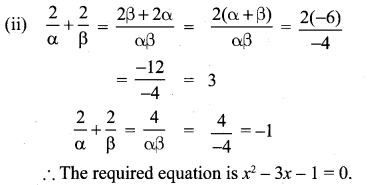(iii) α2β + β2α = αβ(α + β)
= -4(-6) = 24
α2β × β2α = α3β3 = (αβ)3 = (-4)3 = -64
∴ The required equation = x2 – 24x – 64 – 0.

10th Maths Exercise 3.14 Samacheer Kalvi Question 4.
If α, β are the roots of 7x2 + ax + 2 = 0 and if β – α = $$\frac{-13}{7}$$ Find the values of a.
Solution: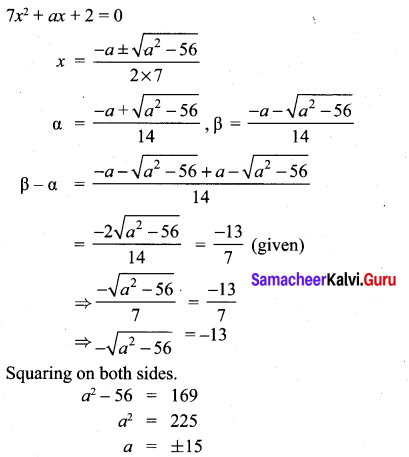10th Maths Exercise 3.14 Solution Question 5.
If one root of the equation 2y2 – ay + 64 = 0 is twice the other then find the values of a.
Solution:
Let one of the root α = 2β
α + β = 2β + β = 3β
Given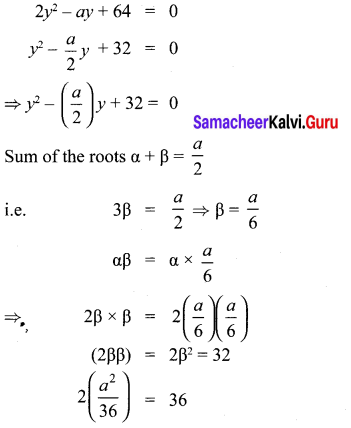a2 = 576
a = 24, -24

Exercise 3.14 Class 10 Samacheer Question 6.
If one root of the equation 3x2 + kx + 81 = 0 (having real roots) is the square of the other then find k.
Solution:
3x2 + kx + 81 = 0
Let the roots be α and α2